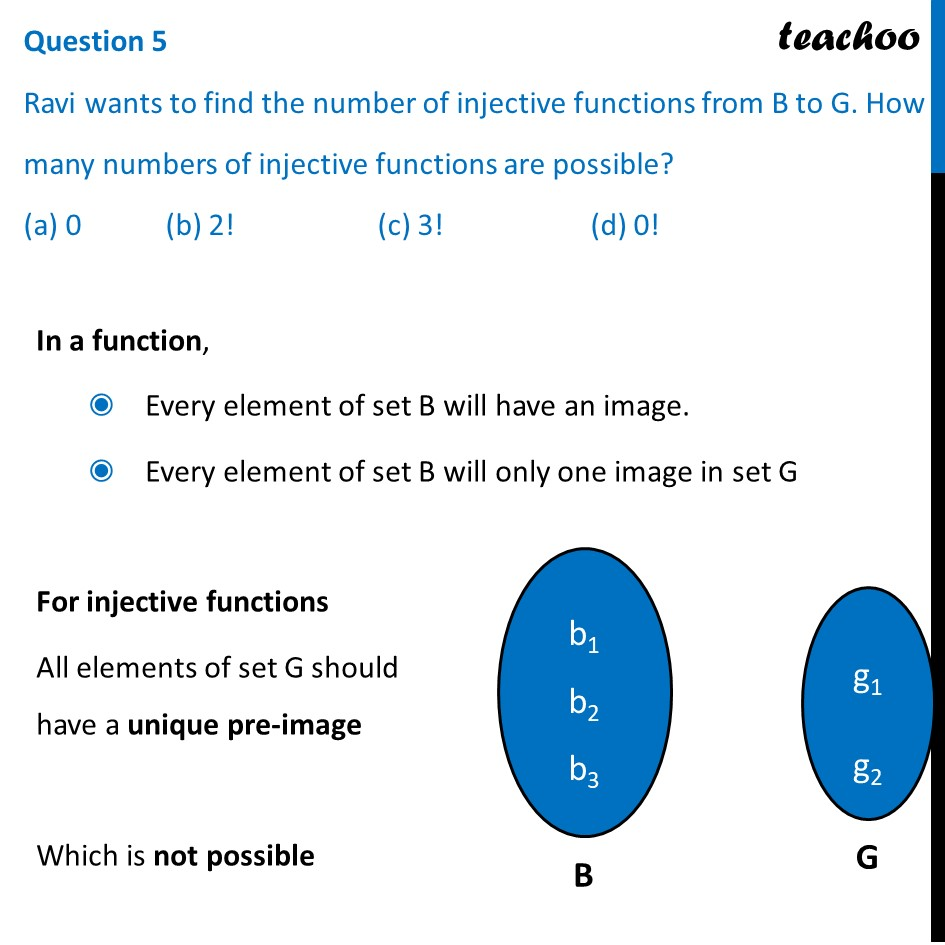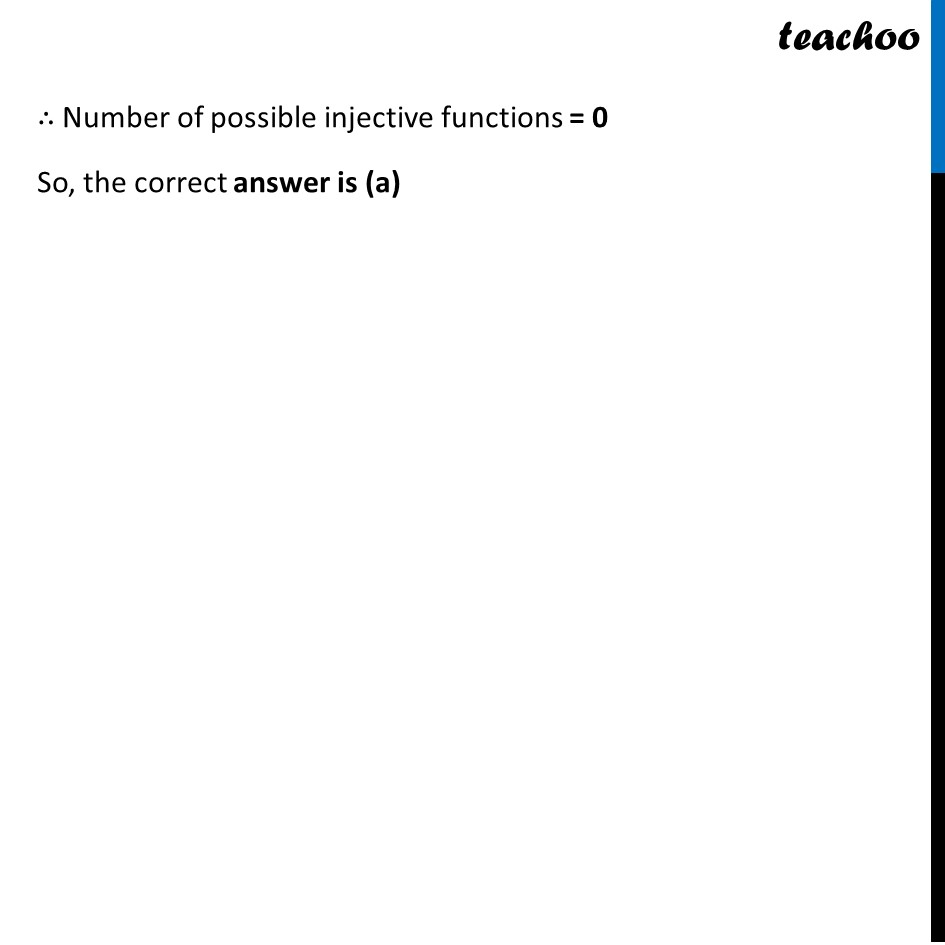##1. Chapter 1 Class 12 Relation and Functions (Term 1)
2. Serial order wise
3. Case Based Questions (MCQ)

Transcript

Question An organization conducted bike race under 2 different categories-boys and girls. Totally there were 250 participants. Among all of them finally three from Category 1 and two from Category 2 were selected for the final race. Ravi forms two sets B and G with these participants for his college project. Let B = {b1, b2, b3} G = {g1, g2} where B represents the set of boys selected and G the set of girls who were selected for the final race. Ravi decides to explore these sets for various types of relations & functions Question 1 Ravi wishes to form all the relations possible from B to G. How many such relations are possible? (a) 26 (b) 25 (c) 0 (d) 23 Given B = {b1, b2, b3} G = {g1, g2} Numbers of Relation from B to G = 2Numbers of elements of B × Number of elements of G = 23 × 2 = 26 So, the correct answer is (a) Question 2 Let R: B → B be defined by R = {(𝑥, 𝑦): 𝑥 and y are students of same sex}. Then this relation R is_______ (a) Equivalence (b) Reflexive only (c) Reflexive and symmetric but not transitive (d) Reflexive and transitive but not symmetric R = {(𝑥, 𝑦): 𝑥 and y are students of same sex}. Check Reflexive Since x and x are of the same sex So, (x, x) ∈ R for all x ∴ R is reflexive Check symmetric If x and y are of the same sex Then, y and x are of the same sex Thus (x, y) ∈ R , and (y, x) ∈ R ∴ R is symmetric Check transitive To check whether transitive or not, If (x, y) ∈ R & (y, z) ∈ R , then (x, z) ∈ R If x and y are of the same sex, And y and z is of the same sex Then, x and z will be of the same sex Thus, for all values of x, y, z (x, y) ∈ R & (y, z) ∈ R , then (x, z) ∈ R ∴ R is transitive Since R is reflexive, symmetric and transitive ∴ R is an Equivalence relation So, the correct answer is (a) Question 3 Ravi wants to know among those relations, how many functions can be formed from B to G? (a) 22 (b) 212 (c) 32 (d) 23 Given B = {b1, b2, b3} G = {g1, g2} So, B has 3 elements, G has 2 elements Numbers of functions from B to G = 2 × 2 × 2 = 23 So, the correct answer is (d) Question 4 Let 𝑅: 𝐵 → 𝐺 be defined by R = {(b1, g1), (b2, g2), (b3, g1)}, then R is__________ (a) Injective (b) Surjective (c) Neither Surjective nor Injective (d) Surjective and Injective Given B = {b1, b2, b3}, G = {g1, g2} And, R = {(b1, g1), (b2, g2), (b3, g1)} So, our relation looks like Injective Injective means one-one Since b1 and b3 have the same image g1 ∴ R is not injective Surjective Surjective means onto Since all elements G has a pre-image ∴ R is surjective So, the correct answer is (b) Question 5 Ravi wants to find the number of injective functions from B to G. How many numbers of injective functions are possible? (a) 0 (b) 2! (c) 3! (d) 0! In a function, Every element of set B will have an image. Every element of set B will only one image in set G For injective functions All elements of set G should have a unique pre-image Which is not possible ∴ Number of possible injective functions = 0 So, the correct answer is (a)

Case Based Questions (MCQ)